# Today’s KenKen

The raging success of Sudoku has created demand for more abstract analytical puzzles, and KenKen seems to fit the bill nicely. Like Sudoku, completing the puzzle requires satisfying a number of overlapping constraints. For KenKen, the rows and columns must have each number only once, and the outlined areas (called cages) must total to the correct number using the specified operator. So a cage marked “10+” must sum to 10, while in one marked “2÷”, the numbers must be a pair that divides to 2.

I find KenKen more interesting than Sudoku, because of the variety of logic that has to be applied to solve one. As an example, here is today’s New York Times KenKen: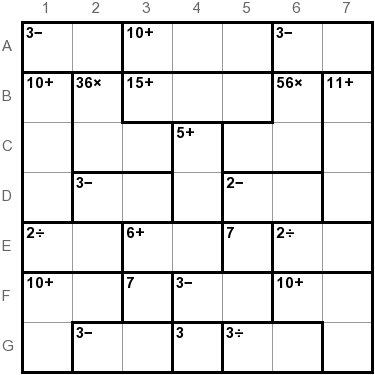I’ll show here step-by-step how I solved it. First we fill in the forced numbers: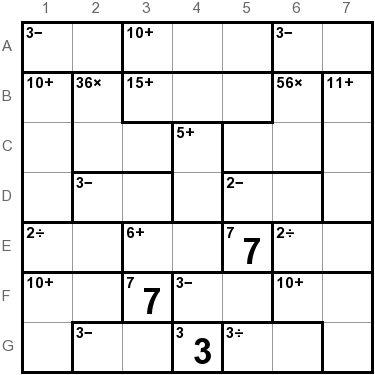At cage C4:D4, we need a sum of 5, which is either 1,4 or 2,3. Column 4 already has a 3, so it must be 1,4, though we don’t know exactly where. Fill in the possibilities: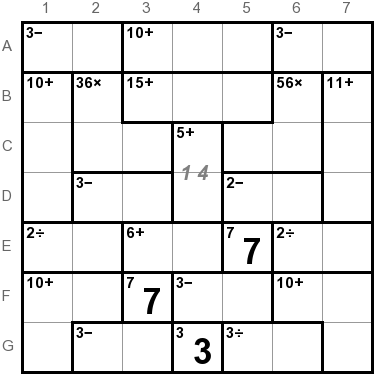Looking at row E, the 5 can’t go in either of the 2÷ cages, so it has to go into the 6+, making it 1,5. Column 4 already has a 1, so we know exactly where they go: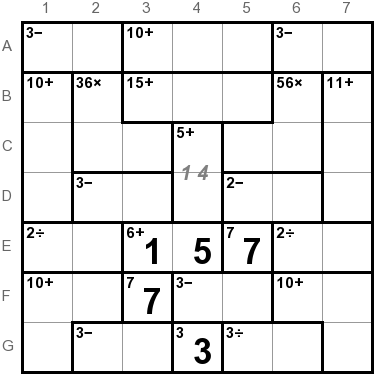G5:G6 is either 1,3 or 2,6, but G4 is already 3, so it must be 2,6: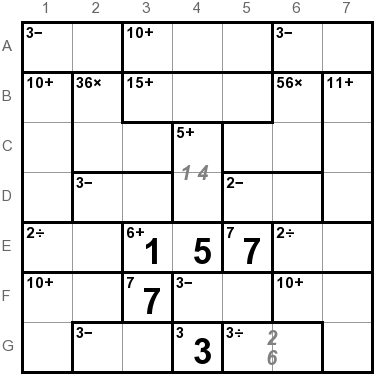Now, consider rows F and G together. The sum of all the cells in both rows must be 56 (2×(1+2+3+4+5+6+7)). Other than F4:F5 and G2:G3, we have a sum of 10+7+10+3+8, or 38. So F4:F5 + G2:G3 is 18. If one of them is 1,4, then the other must sum to 18-5 or 13, making it 5,8, which is impossible, so neither is 1,4. If one of them is 3,6, then the other is also 3,6 but G2:G3 can’t be 3,6 since G4 is already 3. The last remaining possibility is that one of them is 2,5 and the other is 4,7. F4:F5 can’t be 4,7 (since F3 is 7), so it is 2,5, and G2:G3 is 7,4. E4 determines where the 5 will go, and F3 determines where the 7 will go: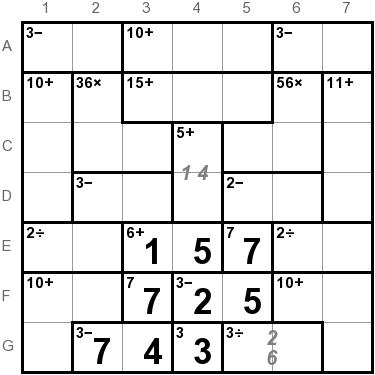In row A, we have a similar arrangement with two 3− cages. Because the entire row sums to 28, and the third cage is a 10+, the two 3− cages together sum to 18, just like we saw in rows F and G. Again 1,4 and 5,8 isn’t a possibility, and because they’re on the same row, we can’t use 3,6 and 3,6. So these cages will also be filled with 2,5 and 4,7, though we don’t know which is which.

That leaves 1,3,6 to fill the 10+ on row A. The 1 has to go in column 5 since columns 3 and 4 already have 1’s. Column 4 has a 3, so A4 gets the 6, and A3 gets the 3: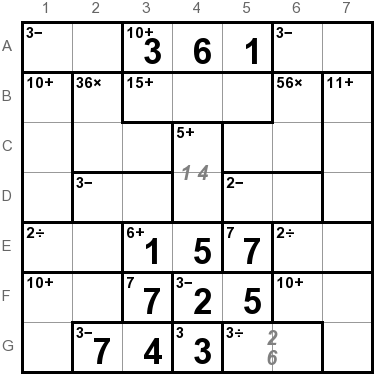The last number in column 4 is the 7 for B4. The 56× cage needs a 7, which can’t go in row B or column 5, so it goes in C6: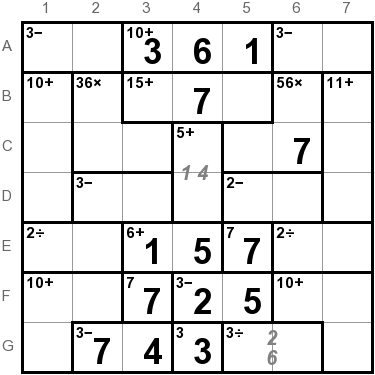Now comes some complicated logic. Look at rows B, C, and D. All togther, their cells have to sum to 84. We know the 56× cage has to be 7,2,4, so the only cages we don’t know the sums of are 36×, 3−, and 2−. The others sum to 54.

There are three possibilities for the 36× cage: 3,4,3 or 6,2,3 or 6,1,6. Let’s consider them in turn:

• If 36× is 3,4,3, then the 3− and 2− cages have to sum to 20, which isn’t possible since the sum of a 3− must be odd and the sum of a 2− must be even, which sum to odd.
• If 36× is 6,2,3, then the 3− and 2− cages sum to 19. There are three ways to do that, none of which are allowed:
• 7,4 and 5,3: won’t work, because columns 2 and 3 already have 7’s.
• 6,3 and 6,4: that would put two 6’s in row D.
• 5,2 and 7,5: two 5’s in row D.
• So 36× must be 6,1,6. Let’s look at the possibilities for the 3− and 2−:
• 7,4 and 4,2: nope, two 4’s in row D.
• 6,3 and 5,3: nope, two 3’s in row D.
• 5,2 and 6,4: that’s valid!
• 4,1 and 7,5: nope, columns 5 and 6 both have 7’s already.

So there’s only one way to complete the 3− and 2− in row D, and we know the solution to the 36×. Also, the possibilites for the 2− cage force the positions of the 1 and 4 in column 4: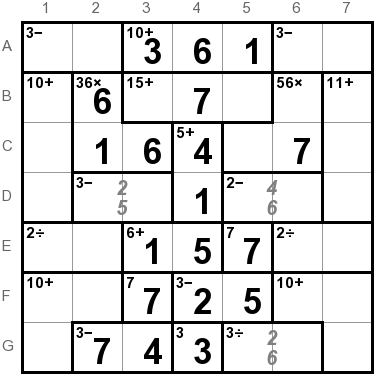Column 3 is almost completed. D3 is either 2 or 5, so B3 is either 5 or 2. If it’s 2, then B5 must be 6, but column 5 will get a 6 from either D5 or G5, so B3 must be 5, and D2 and D3 are determined also: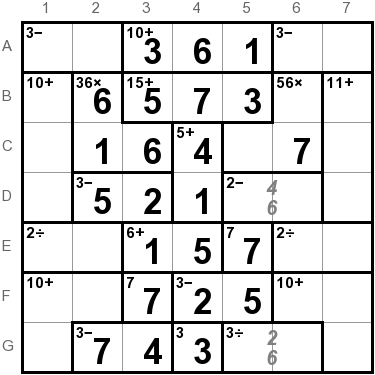The 56× cage has to be 7,2,4 and we now have enough squares filled in to see where they go. These then fix the positions of two cages on rows D and G: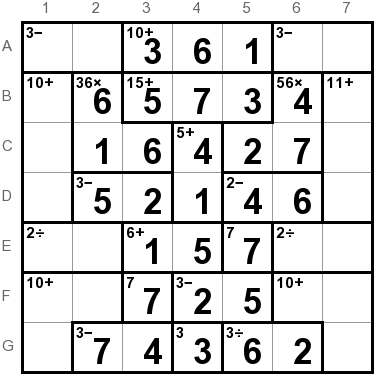Returning to the two 3− cages in row A: they are 2,5 and 4,7. With column 6 more filled in, we can see that A6:A7 has to be 5,2, and A1:A2 is 7,4: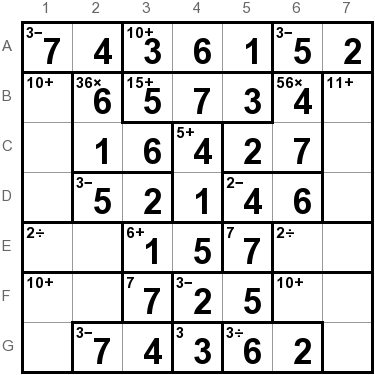The two 2÷ cages in row E will be 2,4 and 3,6. Neither 2 or 4 can go in column 6, so those cages can be filled in, along with the last remaining numbers in columns 2 and 6: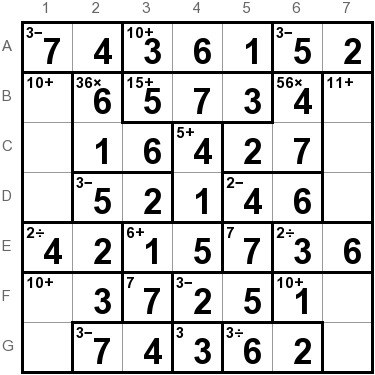Now our rows have only two numbers each remaining. In each row we can consider the two missing numbers, and place them in the column that doesn’t already have it: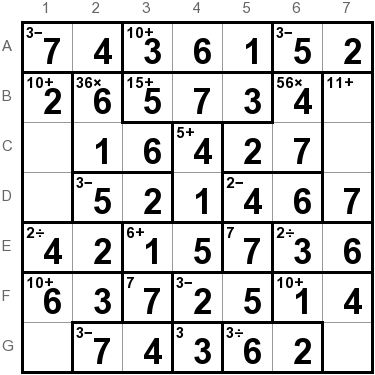And the rest is easy: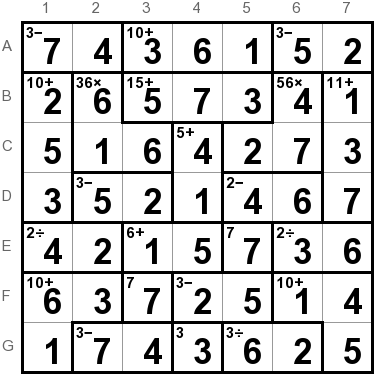KenKen gets old pretty fast. The best puzzle I've found yet is called Killer Sudoku: http://search.barnesandnoble.com/The-Official-Book-of-Killer-Sudoku/Steve-Arons/e/9780452287952. Only the one book that I know of, sad to say.You may be interested in Simon Tatham's Portable Puzzle Collection: http://www.chiark.greenend.org.uk/~sgtatham/puzzles/

There are some quite clever puzzles in that collection, but I don't think they extend to quite the depth that KenKen appears to from your description. Nevertheless, they're great for a short break!Great lesson. KenKen can get old fast, unless you go out and try to find some really nasty ones.

I've made some free videos about how to solve a basic 4x4 Kenken, as well as an advanced 9x9 KenKen in which the operation signs are not given ( a fairly nasty one ).

You can check them out at:

http://mathmojo.com/kenken

Keep up to good blogging.

Brian (a.k.a. Professor Homunculus at MathMojo.com )Try ZenKen81 at the iTunes app store. It's a nice version of KenKen that automatically generates puzzles from 4x4 up to 9x9. You can set your own level of difficulty by selecting the mix of cage sizes, from two squares per cell up to five cells per cage. You can also choose which, if any, arithmetic operators appear in the clues. The full description is at:

http://benrondanpuzzles.com/The 10 % that are capable of solving this level of KenKen minus another 8% of the 10% that simply don't have the time to solve this great puzzle, will keep KenKen from being a huge success like Sudoku!

The go to puzzle to follow in the footsteps of Sudoku is Str8ts. Everyone can solve this puzzle and it is a lot similar in characteristics to Sudoku than any other puzzle! Try it out on the web www.str8ts.com or on the iPhone.If you're looking for more KenKen puzzles, then have a look at,
They also have Sudoku, Nonograms, Hitori and loads of other types of puzzles.If you're looking for more KenKen puzzles or brain training games, then have a look at,
https://brainapps.io
They also have Sudoku, Memory Matrix, IQ test and loads of other types of puzzles.